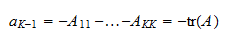StatLect

# Characteristic polynomial

The characteristic polynomial of a square matrix is the polynomial that has the eigenvalues of the matrix as its roots.

We have already introduced the characteristic polynomial in the lecture on eigenvalues. Here we study its properties in greater detail.## Definition

Here is a definition.

Definition Letbe amatrix. The characteristic polynomial ofis the polynomialwhereis theidentity matrix.

Here is a simple example.

Example Define thematrixThen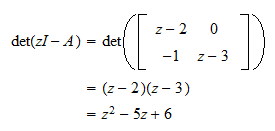Therefore, the characteristic polynomial ofis## Degree

The characteristic polynomial is monic (i.e., the coefficient of its highest power is) and its degree is equal to the dimension of the matrix.

Proposition Letbe amatrix. The characteristic polynomial ofis a monic polynomial of degree.

Proof

This proposition can be proved by using the definition of determinant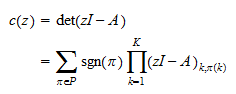whereis the set of all permutations of the firstnatural numbers. Thus,is a sum of polynomials of the formThe polynomial of this form having the highest degree is that in which all the factorsare diagonal elements of. It corresponds to the permutationin which thenatural numbers are sorted in increasing order. The parity ofis even and its sign isbecause it does not contain any inversion (see the lecture on the sign of a permutation). Thus, the summand having the highest degree is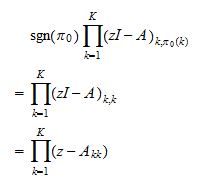which has degreeand is monic. All the other summands have degree less than. Therefore,has degreeand is monic.

## Constant

Being a monic polynomial of degree, the characteristic polynomial can be written as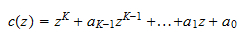Hence,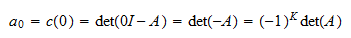where the last equality is a consequence of the properties of the determinant.

## Trace

We also have the following property:whereis the trace of. The proof of this fact can be found in a solved exercise at the end of this lecture.

## Fundamental theorem of algebra

By the Fundamental Theorem of Algebra, a monic polynomial of degreewhose coefficients are complex can be factored into the product oflinear factors (revise the lecture on polynomials if you are puzzled). As a consequence, the characteristic polynomial can be written as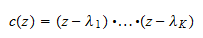whereare the roots of, that is, the values such that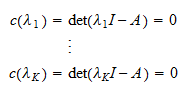In other words, the roots of the characteristic polynomial are the eigenvalues of.

## Solved exercises

Below you can find some exercises with explained solutions.

### Exercise 1

Prove the above claim thatSolution

We have seen above that the characteristic polynomialis a sum of polynomials: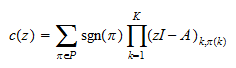whereis a permutation of the firstnatural numbers. We have already seen that there is only one summand that contains aterm, corresponding to the permutation such that. This is also the only summand that contains aterm because, as soon as we invert the order of two numbers in the permutation, two diagonal terms drop out of the productHence, we just need to find the coefficient ofin the productBy expanding the product, we can see that all theterms are of the formand there aresuch terms (for). Therefore,